Next: About this document ... Up: lab_template Previous: lab_template

Subsections

# Applications of exponential and logarithmic functions

## Purpose

The purpose of this lab is to use Maple to study applications of exponential and logarithmic functions. These are used to model many types of growth and decay.

## Background

### Exponential growth

The simple model for growth is exponential growth, where it is assumed thatis proportional to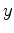. That is,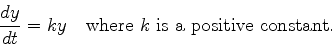Separating the variables and integrating (see section 4.4 of the text), we have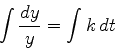so that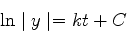In the case of exponential growth, we can drop the absolute value signs around, becausewill always be a positive quantity. Solving for, we obtain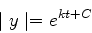which we may write in the form, whereis an arbitrary positive constant.

### Exponential Decay

In a sample of a radioactive material, the rate at which atoms decay is proportional to the amount of material present. That is,whereis a constant. This is the same equation as in exponential growth, except that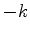replaces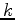. The solution is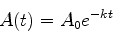where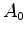is a positive constant. Physically,is the amount of material present at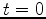.

Radioactivity is often expressed in terms of an element's half-life. For example, the half-life of carbon-14 is 5730 years. This statement means that for any given sample of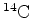, after 5730 years, half of it will have undergone decay. So, if the half-life is of an element Z is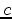years, it must be that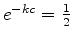, so that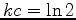and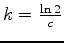.

### Newton's law of cooling

What is usually called Newton's law of cooling is a simple model for the change in temperature of an object that is in contact with an environment at a different temperature. It says that the rate of change of the temperature of an object is proportional to the difference between the object's temperature and the temperature of the environment. Mathematically, this can be expressed as the differential equation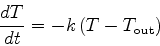whereis the constant of proportionality and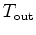is the temperature of the environment. Using a technique called separation of variables it isn't hard to derive the solution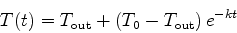where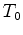is the temperature of the object at.

### Information Diffusion

Information can be thought of as of a physical quantity which can be measured. According to the Gallup Institute, information news diffuses through a fixed adult population of sizeat a rate of time proportional to the number of people who have not heard the news.

Ifis the number of people who have heard the news after t days, then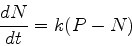The initial condition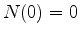yields the solution## Exercises

1. Suppose that the population of a certain bacteria can be modeled by an exponential function. In a particular experiment, the number of bacteria was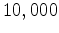at. Four hours later, the bacteria was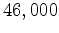. Find the value of the growth constantand use it to predict the number of bacteria that would have been present after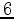hours.
2. Exponential growth can be used to model the growth of a certain kind of investments. Suppose that the value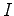of an investment satisfies the differential equation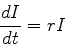where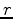is the interest rate. If the interest rate is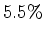per year and you start with an investment of \$12,000, how many years does it take to double? How many years does it take to quadruple? Is there an easy way to answer the second part of this question? Approximately how many years would it take to save a million dollars?
3. A thermometer registered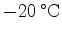outside and then was brought into the house where the temperature was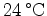. After 5 minutes, it registered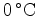. When will it register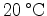?
4. Suppose that 75% of a freshman class of 500 students on a campus heard about the market crash on Wall Street 3 days after it happened. How long will it take for 99% of the freshman to hear the news?Next: About this document ... Up: lab_template Previous: lab_template
Dina J. Solitro-Rassias
2010-09-30